# Change Nickname

Current Nickname:
Search
v2.x
v2.x

# Subgraph Queries

Ultipa offers a rich collection of query functionalities via uQL. With these functionalities, users can explore any graph dataset with ease and celerity, without lengthy and tedious programming language course training. uQL supports:

• Meta-data query (in chapter Meta-Data)
• A-to-B query
• K-Hop query
• Automatic networking
• Template-based query (in chapter Template-Based Query)
• Graph Algorithm
• System management and status query (in Chapter Instance, GraphSet and Property and chapter Task)

The A-to-B query, K-Hop query, automatic networking and spreading to be introduced in this chapter will demonstrate the basic idea of abstracting subgraphs through path-based search.

Examples in this chapter can be validated by running against a test graphset with meta-data information provided: node_info, edge_info

## `ab()`

A-to-B query or shorted as A-B query is to find paths between a pair of nodes by certain filtering rules applied to the meta-data in the path, the search depth can be from 1 to N (for instance, 5 or 10 or 15 or deeper).

Ultipa system uses DFS by default to conduct A-B query, unless being instructed via parameter `shortest()` to search using BFS.

### uQL Components

The uQL components of A-B query:

Type Components
Command `ab()`
Parameter `src(<>)` or `osrc(<>)`, `dest(<>)` or `odest(<>)`, `depth(<>)`, `shortest(<>)`,
`node_filter(<>)`, `edge_filter(<>)`, `path_ascend(<>)`, `path_descend(<>)`, `direction(<>)`, `no_circle()`,
`turbo()`,
`limit(<>)`
Return `select(<>)` or `select_node_properties(<>)` or `select_edge_properties(<>)`

Values of parameter and return:

Name Data Type Specification Description
src int Ultipa ID (1/2) The starting node of the path, does not coexist with `osrc()`
osrc string original ID (2/2) The starting node of the path, does not coexist with `src()`
dest int Ultipa ID (1/2) The ending node of the path, does not coexist with `odest()`
odest string original ID (2/2) The ending node of the path, does not coexist with `dest()`
depth int; range <30 The number of edges in the path;
`depth(N)`: N edges
`depth(:N)`: 1~N edges
`depth(M:N)`: M~N edges
`depth(N).shortest(<>)`: the number of edges of the least-weighted paths within N steps
shortest string edge property (numeric type); / (Optional) To return the least-weighted paths using the specified property as weight factor; /: use 1 as weight factor
node_filter obj Ultipa filter (Optional) The filtering rules that nodes other than the starting/ending node need to satisfy
edge_filter obj Ultipa filter (Optional) The filtering rules that edges need to satisfy
path_ascend string edge property (numeric type) (Optional) To return paths where the specified property ascends along the path
path_descend string edge property (numeric type) (Optional) To return paths where the specified property descends along the path
direction string 'left' or 'right' (Optional) To return paths where all edges are in the same direction along the path, either left or right
no_circle / / (Optional) To dismiss the paths with circles
turbo / / (Optional) To enable turbo mode
limit int >0; -1 (Optional) The maximum number of paths to return; -1: return all the results
select []string comma (,) separated; * (1/2, optional) A list of properties to return; *: return all the properties; does not coexist with `select_node_properties()` or `select_edge_properties()`
select_node_properties []string comma (,) separated; * (1/4, optional) A list of node properties to return; *: return all the node properties; does not coexist with `select()`
select_edge_properties []string comma (,) separated; * (1/4, optional) A list of edge properties to return; *: return all the edge properties; does not coexist with `select()`

Where:

• when `limit()` is not included in the uQL, it's considered as `limit(3)`;
• _id, _from_id and _to_id are the default properties to be returned and need not to be declared in `select()`, `select_node_properties()` and `select_edge_properties()`.

### Examples

Example 1: Find 5 paths between two nodes ( _id = 3 and _id = 72), search depth = 6, return 'type' of nodes and edges

``````ab()
.src(3).dest(72).depth(6)
.limit(5).select(type)
``````

Running Example 1 within Ultipa-Manager will get this: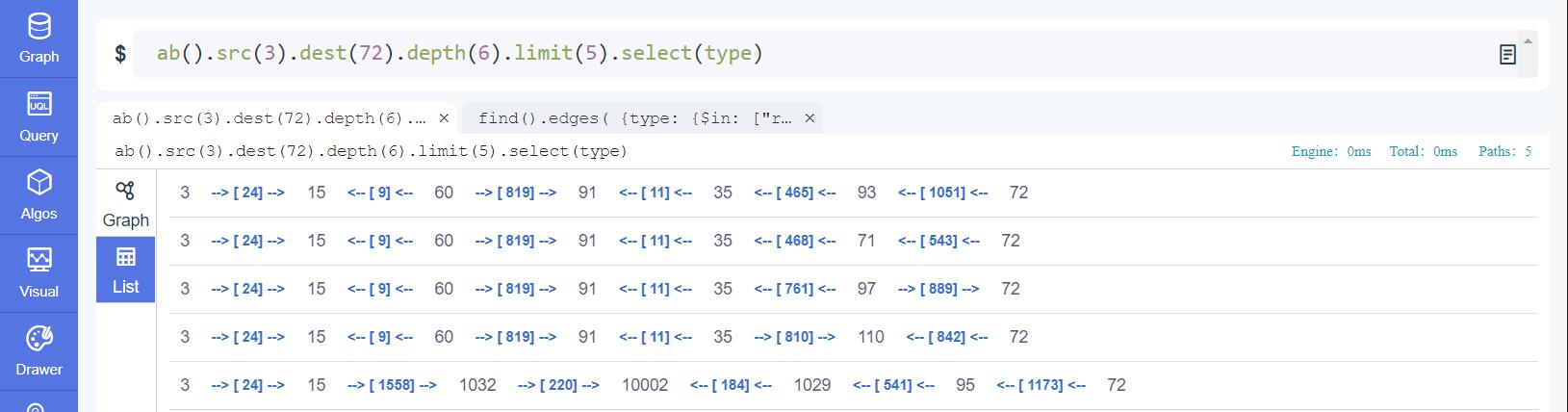Figure: A-B Query with fixed depth

`In the returning paths the edges are labeled with 'type' while the nodes are labeled with '_id' due to that 'type' is not a valid property of node even though 'type' is instructed to be returned for both node and edge.`

Example 2: Same as Example 1, but search depth ≤ 6 and return 'name' and 'type' of nodes and 'type' of edges

``````ab()
.src(3).dest(72).depth(:6)
.limit(5)
.select_node_properties(name, type)
.select_edge_properties(type)
``````

Running Example 2 within Ultipa-Manager will get this: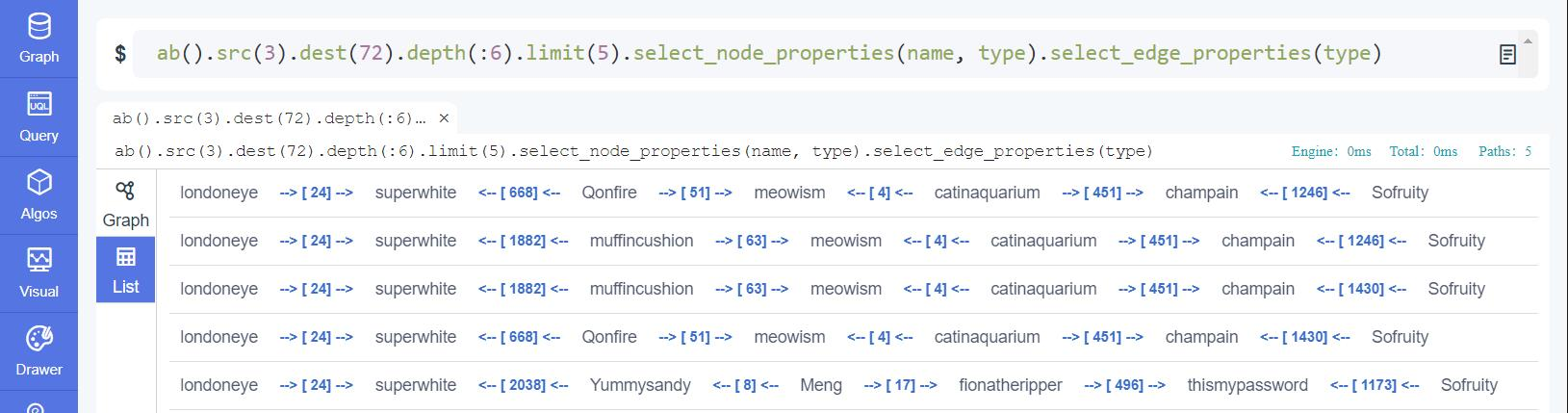Figure: A-B Query with depth range

`Compare the difference on the length of paths returned under fixed depth and depth range`

Example 3: Same as Example 2, but return the shortest paths (least-weighted by using 1 as weight factor)

``````ab()
.src(3).dest(72).depth(6).shortest()
.limit(5).select(name, type)
``````

`When using shortest(), no colon (:) used in the depth setting.`

Example 4: Same as Example 2, but search depth between 2 ~ 4 and the in-between nodes are all accounts of users born in the 1980s

``````ab()
.src(3).dest(72).depth(2:4)
.node_filter( {type: "account", year: {\$bt:[1980,1989]} } )
.limit(5).select(name, type)
``````

`For detailed instructions on Ultipa filters please review the content of chapter Filter Mechanism.`

Example 5: Same as Example 4, but replace the node filter with edge filter that requires all the edges to be 'response' type

``````ab()
.src(3).dest(72).depth(2:4)
.edge_filter( {type: "response"} )
.limit(5).select(name, type)
``````

Example 6: Based on solution of Example 5, tune the parameters to have each user responding to its previous user in 'timestamp' ascending fashion

``````ab()
.src(3).dest(72).depth(2:4)
.edge_filter({type: "response"})
.path_ascend(timestamp).direction(left)
.limit(5).select(name, type)
``````

Example 7: Based on solution of Example 2, turn turbo mode on

``````ab()
.src(3).dest(72).depth(:6)
.turbo()
.limit(5).select(name, type)
``````

`Note: TURBO mode is a patent-pending way of accelerating path finding, being useful especially when encountering hot-spot node(s). By a loose definition, hot-spot nodes are super nodes that have many more 1-hop neighbors than average, like nodes with 100 thousand 1-hop neighbors for instance. By applying turbo(), there is an opportunity to achieve 50% to 300% of performance improvement on average.`

## `khop()`

K-Hop query is to find neighbor nodes that a starting node can reach at K hops, with K being the least number of hops required. For instance, to find a node's 3-hop neighbors is to find the collection of all nodes that are at least 3-hop away from it.

Ultipa system uses BFS to guarantee that the paths for reaching the neighbors are the shortest, and the k-hop neighbors will only appear at k hops, neither farther nor nearer; when a neighbor appears more than once at its specific hop via different shortest paths, no duplicated results will be returned. These principles of Ultipa K-Hop query are recapped as:

• BFS
• De-duplication

`Although knowing that DFS does NOT tell whether a path is a valid shortest path or not, a few graph systems in the market are implementing K-hop in DFS way for the sake of query speed. Users pursuing authentic K-hop functionalities should be aware of the incorrectness and redundancy of the query result from these kinds of DFS derived K-hop implementations.`

### uQL Components

The uQL components of K-Hop query:

Type Components
Command `khop()`
Parameter `src(<>)` or `osrc(<>)`, `depth(<>)`,
`node_filter(<>)`, `edge_filter(<>)`, `direction(<>)`,
`limit(<>)`
Return `select(<>)`, total number of results

Values of parameter and return:

Name Data Type Specification Description
src int Ultipa ID (1/2) The starting node of the shortest path, does not coexist with `osrc()`
osrc string original ID (2/2) The starting node of the shortest path, does not coexist with `src()`
depth int; range <30 The number of edges in the shortest path;
`depth(N)`: N edges
`depth(:N)`: 1~N edges
`depth(M:N)`: M~N edges
node_filter obj Ultipa filter (Optional) The filtering rules that nodes other than the starting node need to satisfy
edge_filter obj Ultipa filter (Optional) The filtering rules that edges need to satisfy
direction string 'left' or 'right' (Optional) To return paths where all edges are in the same direction along the path, either left or right
limit int >0; 0; -1 (Optional) The maximum number of ending nodes to return; -1: return all the results, 0: only reutrn the total number of results found
select []string comma (,) separated; * (Optional) A list of properties to return; *: return all the properties

Where:

• when `limit()` is not included in the uQL, it's considered as `limit(3)`;
• _id is the default property to be returned and need not be declared in `select()`.

### Examples

Example 1: Find 1-hop neighbors of movie The Shawshank Redemption ( _id = 1009 ), limit to 10 results

``````khop()
.src(1009).depth(1)
.limit(10)
``````

Example 2: Same as Example 1, but 2-hop neighbors with 'name' and 'type' returned

``````khop()
.src(1009).depth(2)
.limit(10).select(name,type)
``````

Example 3: Based on solution of Example 2, all edges need to be 'filmedIn' type

``````khop()
.src(1009).depth(2)
.edge_filter( {type: "filmedIn"} )
.limit(10).select(name,type)
``````

Example 4: Same as Example 2, but 2-to-3 hop neighbors of U.S. ( _o = m_1000 )

``````khop()
.osrc("m_1000").depth(2:3)
.limit(10).select(name,type)
``````

Example 5: Based on solution of Example 1, compare the total number of results at 2-hop, 3-hop and 2-to-3 hop; use limit(0) to omit the information of neighbors

``````khop().src(1009).depth(2).limit(0);
khop().src(1009).depth(3).limit(0);
khop().src(1009).depth(2:3).limit(0)
``````

Running Example 5 within Ultipa-Manager will get these: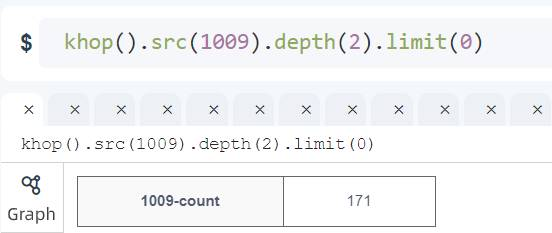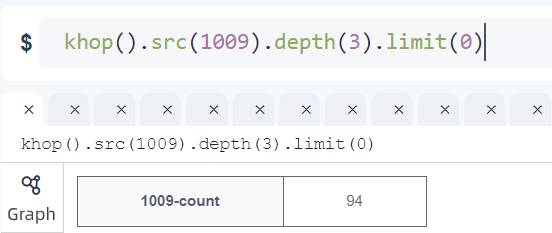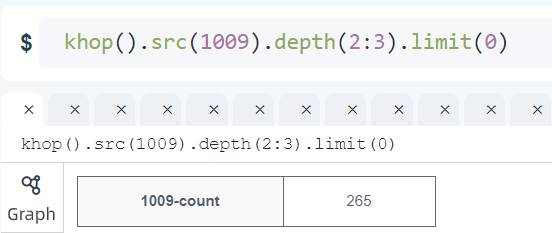Figure: K-Hop Query with returned values at different hops

The screenshots from above shows:

• How limit(0) simplifies the system return
• How range-specified K-hop is performed, ie., depth(2:3) = depth(2) + depth(3)

## `autoNet()`

The beauty of graph computing and analytics is that when large amounts of data are forming rather complex networks, a subgraph can help to quickly identify specific data connections and make things explainable (a.k.a. white-box graph operations).

Automatic networking is a subgraph extraction functionality more efficient than A-B query, allowing multiple starting/ending nodes to be defined and fewer parameters to be set.

Automatic networking works in 2 modes:

1. given N nodes, find the paths between any two of them, pair by pair. In an ideal situation, this is equivalent to finding `C(N,2)` * `limit(X)` paths;
2. given N nodes, find the paths to another M nodes, which is equivalent to finding `N` * `M` * `limit(X)` paths.

### uQL Components

The uQL components of automatic networking:

Type Components
Command `autoNet()`
Parameter `srcs(<>)`, `dests(<>)`, `depth(<>)`, `shortest()`,
`node_filter(<>)`, `edge_filter(<>)`, `no_circle()`,
`turbo()`,
`limit(<>)`
Return `select(<>)` or `select_node_properties(<>)` or `select_edge_properties(<>)`

Values of parameter and return:

Name Data Type Specification Description
srcs []int Ultipa ID The starting nodes of the path
dests []int Ultipa ID (Optional) The ending nodes of the path
depth int; range <30 The number of edges in the path;
`depth(N)`: N edges
`depth(:N)`: 1~N edges
`depth(M~N)`: M~N edges
`depth(N).shortest(<>)`: the number of edges of the shortest paths within N steps
shortest / / (Optional) To return the shortest paths
node_filter obj Ultipa filter (Optional) The filtering rules that nodes other than the starting/ending node need to satisfy
edge_filter obj Ultipa filter (Optional) The filtering rules that edges need to satisfy
no_circle / / (Optional) To dismiss the paths with circles
turbo / / (Optional) To enable turbo mode
limit int >0; -1 (Optional) The maximum number of paths between each pair of starting/ending node to return; -1: return all the paths between each node pair
select []string comma (,) separated; * (1/2, optional) A list of properties to return; *: return all the properties; does not coexist with `select_node_properties()` or `select_edge_properties()`
select_node_properties []string comma (,) separated; * (1/4, optional) A list of node properties to return; *: return all the node properties; does not coexist with `select()`
select_edge_properties []string comma (,) separated; * (1/4, optional) A list of edge properties to return; *: return all the edge properties; does not coexist with `select()`

Where:

• when `dests()` is not included in the uQL, it's considered as the first function mode, otherwise the second;
• when `limit()` is not included in the uQL, it's considered as `limit(3)`;
• _id, _from_id and _to_id are the default properties to be returned and need not to be declared in `select()`, `select_node_properties()` and `select_edge_properties()`.

### Examples

Example 1: Form a network using nodes ( _id = [91,1012,1020,20018] ), search depth = 4, limit to 2 paths between each pair of nodes

``````autoNet()
.srcs(91, 1012, 1020, 20018).depth(4)
.limit(2).select(*)
``````

Above uQL returns maximum (4 * 3) / 2 * 2 = 12 paths. Running Example 1 within Ultipa-Manager will get these: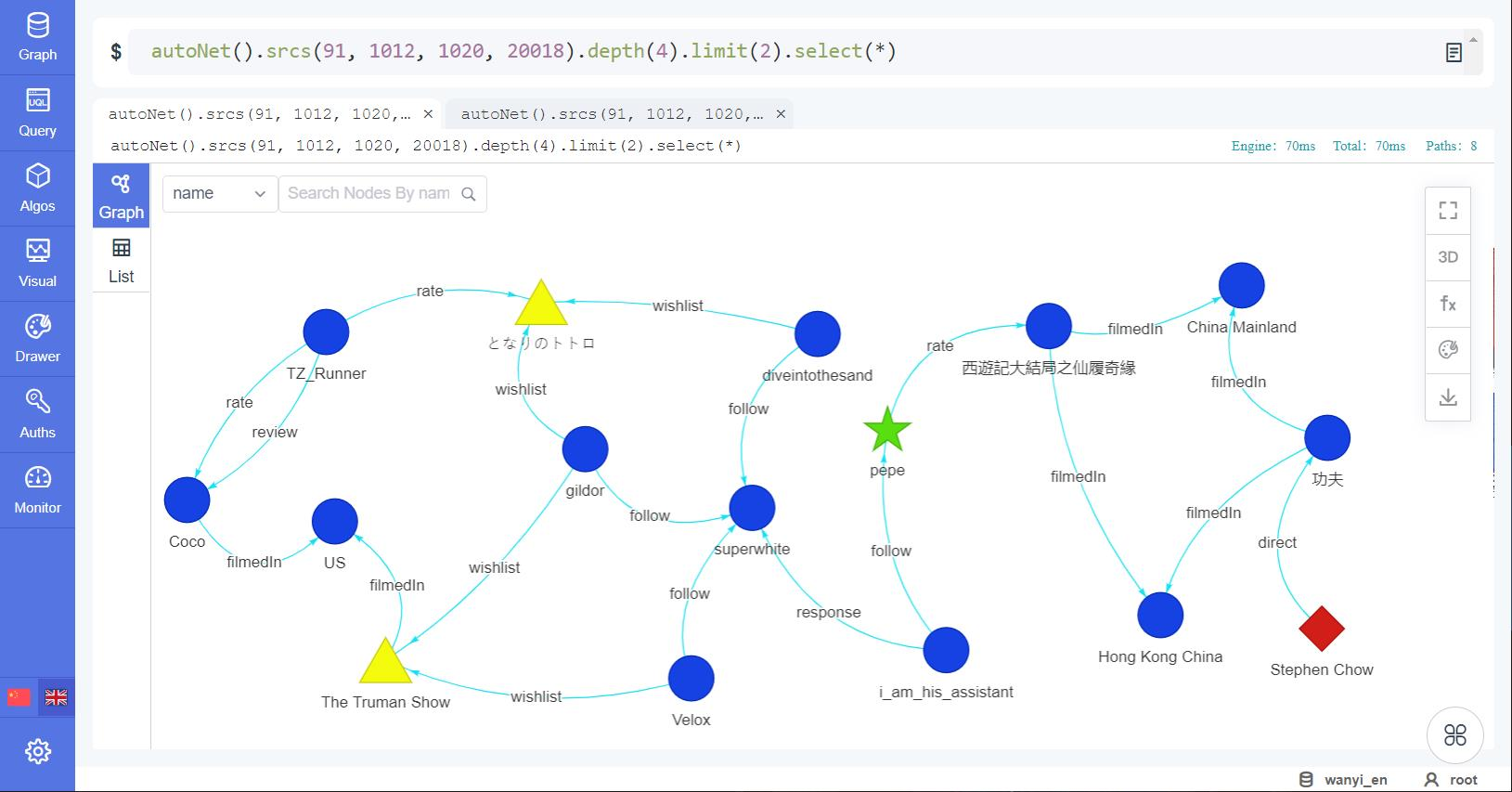Figure: autoNet() Query Results in 2D Mode

Graph can be very illustrative and helpful with interpretability yet being misleading, because when a subgraph is formed, what you see may NOT be what it really is! Ultipa provides List Mode to complement the Graph Mode so that you can have the best of both worlds handy. See below results in List Mode: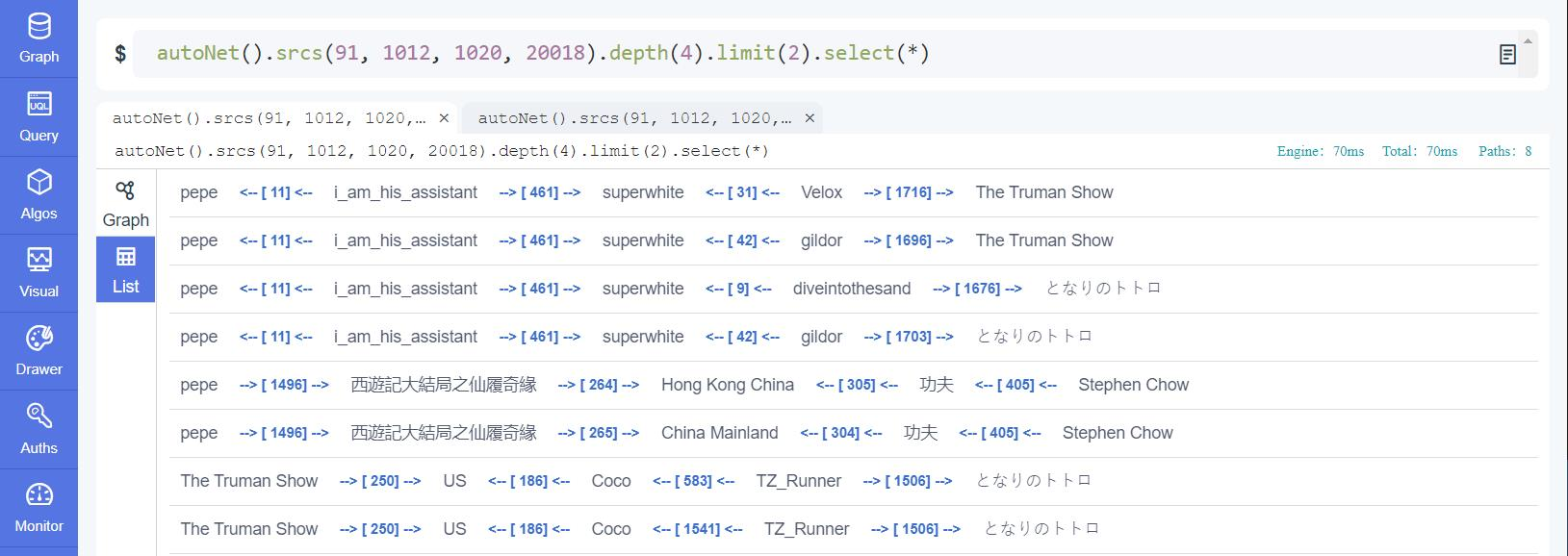Figure: autoNet() Query Results in List Mode

Example 2: Form a network between 2 sets of nodes: [91,20018] and [1012,1020], set depth ≤ 5, limit to 2 paths between each pair of nodes, having no circles.

``````autoNet()
.srcs(91, 20018).dests(1012, 1020).depth(:5)
.no_circle()
.limit(2).select(*)
``````

Above uQL returns maximum (2 * 2) * 2 = 8 paths.

## `spread()`

Automatic spreading is to spread from a specified node in a BFS fashion for its neighborhood information.

Automatic spreading has parameters mostly similar to that of K-Hop query, but with simplified `depth(<>)` setting and enriched returns including all the meta-data in the shortest paths.

### uQL Components

The uQL components of automatic spreading:

Type Components
Command `spread()`
Parameter `src(<>)` or `osrc(<>)`, `depth(<>)`,
`node_filter(<>)`, `edge_filter(<>)`, `direction(<>)`,
`limit(<>)`
Return `select(<>)` or `select_node_properties(<>)` or `select_edge_properties(<>)`

Values of parameter and return:

Name Data Type Specification Description
src int Ultipa ID (1/2) The starting node of the shortest path, does not coexist with `osrc()`
osrc string original ID (2/2) The starting node of the shortest path, does not coexist with `src()`
depth int <30 The maximum depth to spread
node_filter obj Ultipa filter (Optional) The filtering rules that nodes other than the starting node need to satisfy
edge_filter obj Ultipa filter (Optional) The filtering rules that edges need to satisfy
direction string 'left' or 'right' (Optional) To return paths where all edges are in the same direction along the path, either left or right
limit int >0; -1 (Optional) The maximum number of ending nodes to return; -1: return all the results
select []string comma (,) separated; * (1/2, optional) A list of properties to return; *: return all the properties; does not coexist with `select_node_properties()` or `select_edge_properties()`
select_node_properties []string comma (,) separated; * (1/4, optional) A list of node properties to return; *: return all the node properties; does not coexist with `select()`
select_edge_properties []string comma (,) separated; * (1/4, optional) A list of edge properties to return; *: return all the edge properties; does not coexist with `select()`

Where:

• when `limit()` is not included in the uQL, it's considered as `limit(3)`;
• _id, _from_id and _to_id are the default properties to be returned and need not to be declared in `select()`, `select_node_properties()` and `select_edge_properties()`.

### Examples

Example 1: Spread from node of country Iceland ( _id = 10009 ) within 2 steps, return up to 100 nodes together with edges, return name and type (of nodes/edges)

``````spread()
.src(10009).depth(2)
.limit(100).select(name, type)
``````

Running Example 1 within Ultipa-Manager will get these: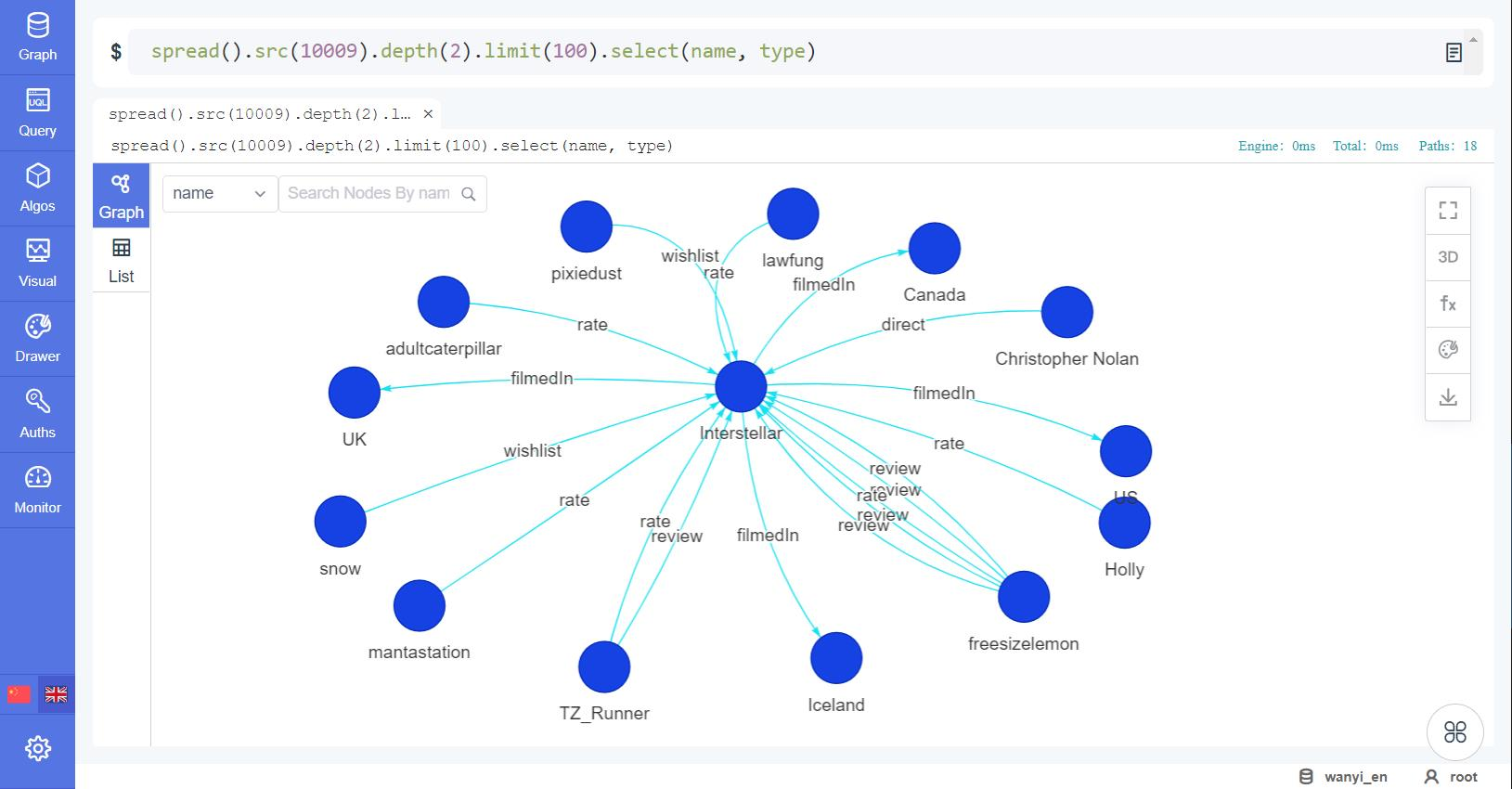Figure: spread() Query Results in 2D Mode

When not being truncated by `limit(<>)`, the nodes returned by `spread()...depth(N)` are same with those returned by `khop()...depth(:N)`. See below screenshot of a `khop()` command which is an equivalent of the solution of Example 1: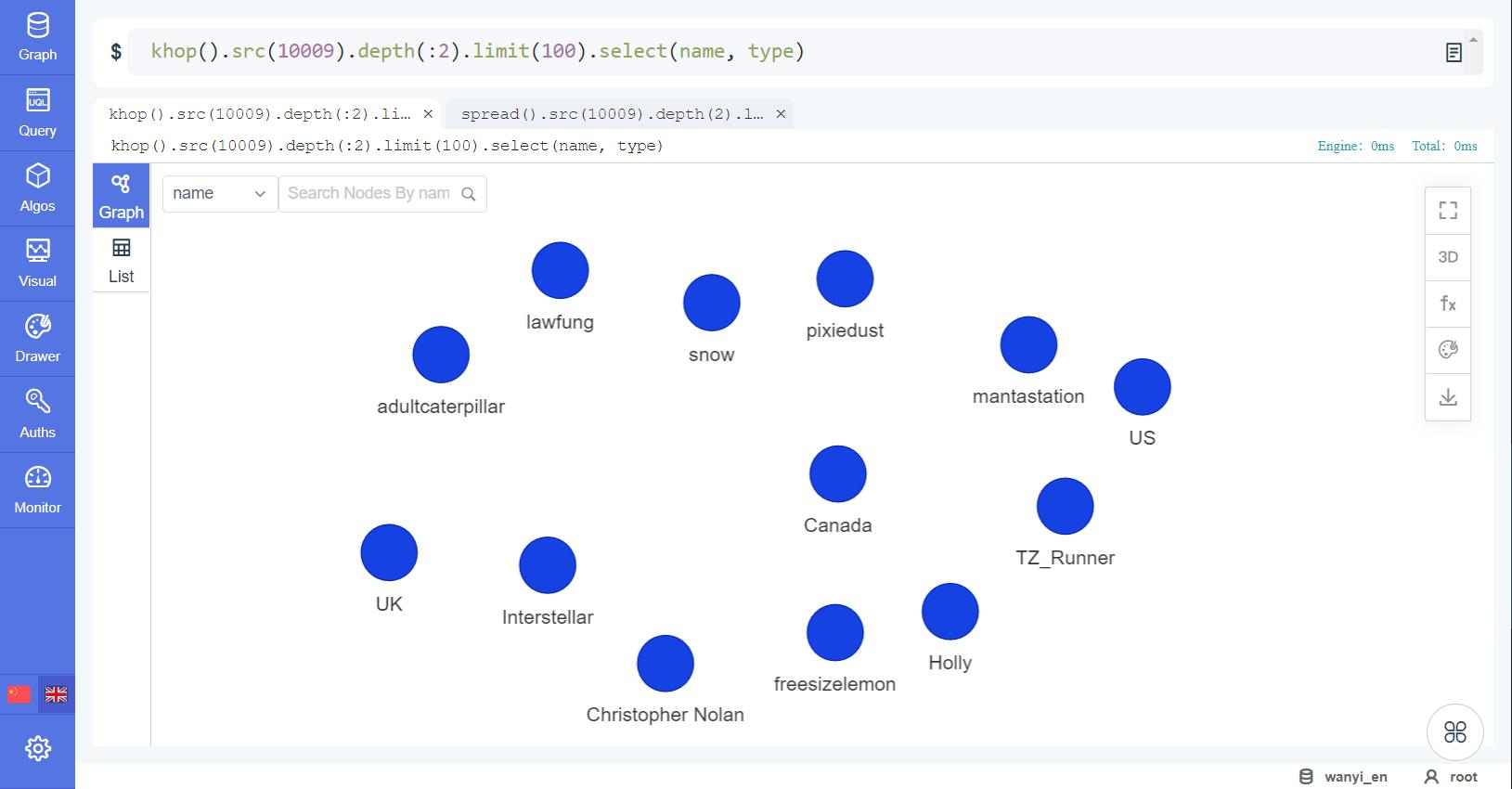Figure: khop() Query Results compared to spread()

Example 2: Spread from node of movie Léon ( _id = 1004 ) within 5 steps via left directed edges, return up to 100 nodes together with edges

``````spread()
.src(1004).depth(5)
.direction(left)
.limit(100).select(name, type)
``````

Automatic spreading can be very useful in many scenarios. For instance, in the explainable AI or recursive/graph computing, it can help traverse and explore the data in a rather controlled and white-box way, therefore making things explainable.